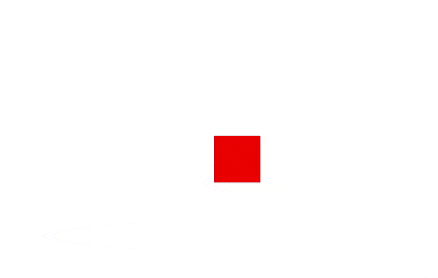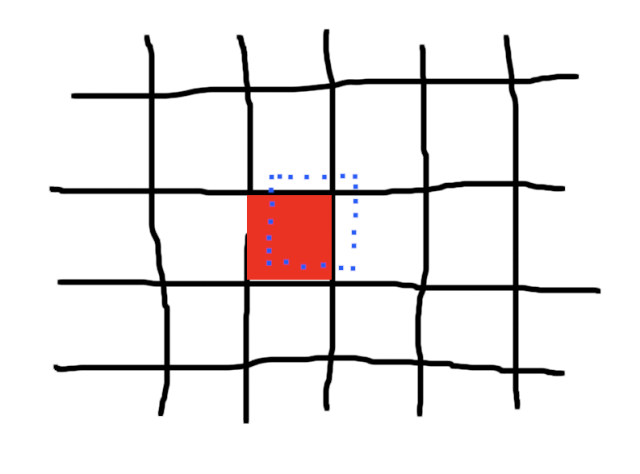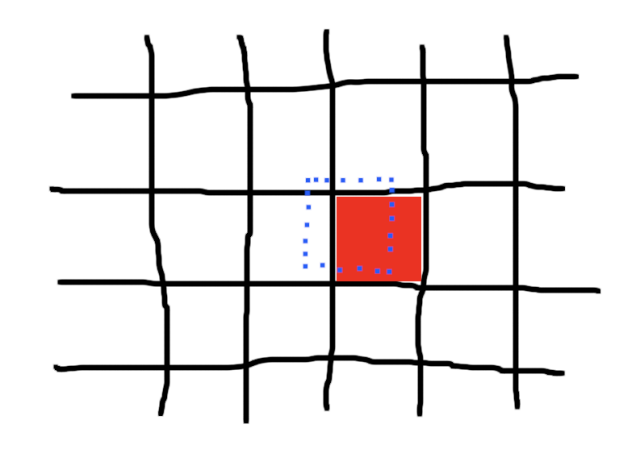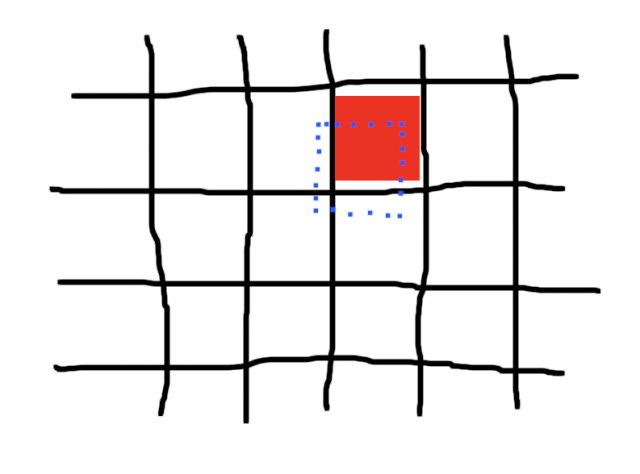# Mobile puzzle game using Titanium SDK (part 2)

Check part 1 first in case you’ve missed that!

In the second part we will generate a grid movement.## Current code

Your current files should look like this

```				```
var startX = 0;
var startY = 0;
var isMoving = false;
var activeItem = \$.view;

function onTouchstart(e) {
startX = e.x;
startY = e.y;
isMoving = true;
}

function onTouchmove(e) {
if (isMoving) {
let convertedPoint = activeItem.convertPointToView({
x: e.x,
y: e.y
}, activeItem.parent);

activeItem.left = convertedPoint.x - startX;
activeItem.top = convertedPoint.y - startY;
}
}

function onTouchend(e) {
isMoving = false;
}

\$.index.open();

```
```
```				```
<Alloy>
<Window onTouchmove="onTouchmove" onTouchend="onTouchend">
<View id="view" onTouchstart="onTouchstart"/>
</Window>
</Alloy>

```
```
```				```
"#view" : {
width: 40,
height: 40,
backgroundColor: 'red'
}

```
```

## Grid movement

The idea is that we have define a grid size and only move the view if our x/y position is greater than half of the grid size.Create two variables to store the grid size and half of it (then we don’t need to calculate it all the time)

```				```
const gridSize = 40; // size of our grid
const halfGridSize = gridSize * 0.5;
```
```

Then we update our `onTouchmove` method. First store the position that we assigned to the activeItem before:

```				```
let posX = convertedPoint.x - startX;
let posY = convertedPoint.y - startY;
```
```

Next we need to see if our `posX`/`posY` is before or after `halfGridSize`. To do that we use the module operator:

```				```
let modX = posX % gridSize;    // value from 0 to gridSize
let modY = posY % gridSize;    // value from 0 to gridSize

// check (modX > halfGridSize)
// check (modY > halfGridSize)
```
```

if this is `true` we move one gridSize right, if `false` we stay at our previous grid position.

To stay at the grid location we simply subtract the modulo value from `posX`. To jump to the next grid position we add `gridSize`. The full movement code will look like this now:

```				```
let targetX = modX > halfGridSize ? posX - modX + gridSize : posX - modX;
let targetY = modY > halfGridSize ? posY - modY + gridSize : posY - modY;
```
```

Now we use this value and assign it to our current item

```				```
activeItem.left = targetX;
activeItem.top = targetY;
```
```

When dragging the view it will now move in our grid structure.## Final code

```				```
var startX = 0;
var startY = 0;
var isMoving = false;
var activeItem = \$.view;
const gridSize = 40;
const halfGridSize = gridSize * 0.5;

function onTouchstart(e) {
startX = e.x;
startY = e.y;
isMoving = true;
}

function onTouchmove(e) {
if (isMoving) {

let convertedPoint = activeItem.convertPointToView({
x: e.x,
y: e.y
}, activeItem.parent);

let posX = convertedPoint.x - startX;
let posY = convertedPoint.y - startY;

let modX = posX % gridSize;
let modY = posY % gridSize;

let targetX = modX > halfGridSize ? posX - modX + gridSize : posX - modX;
let targetY = modY > halfGridSize ? posY - modY + gridSize : posY - modY;

activeItem.left = targetX;
activeItem.top = targetY;
}
}

function onTouchend(e) {
isMoving = false;
}

\$.index.open();

```
```

## Like the content?

If you like the tutorials, plug-ins or want to see more please consider becoming a Github sponsor.

Content